# WLAN HDL LDPC Encoder

This example shows how to implement a WLAN LDPC encoder using Simulink® blocks that are optimized for HDL code generation. This example supports the IEEE® 802.11n™, 802.11ac™, 802.11ax™, and 802.11ad™ standards. The WLAN LDPC Encoder block in this example works in conjunction with the WLAN LDPC Decoder block. To verify the behavior of the WLAN LDPC Encoder block, compare the output of the block with the output of the ldpcEncode function.

### Introduction

The IEEE 802.11n (also known as Wi-Fi™ 4) [ 1 ], IEEE 802.11ac (also known as Wi-Fi 5) [ 1 ] and IEEE 802.11ax (also known as Wi-Fi 6) [ 2 ] standards use convolutional codes for forward error correction (FEC). Due to the advantages over convolutional codes, the standards use low-density parity-check (LDPC) codes. These standards define quasi-cyclic (QC) LDPCs with codeword block lengths of 648, 1296, and 1944 and subblock sizes, also known as expansion factors, of 27, 54, and 81, respectively. For each codeword block length, the standard defines code rates of 1/2, 2/3, 3/4, and 5/6.

The IEEE 802.11ad [ 3 ] standard defines a QC LDPC with a codeword block length of 672 and a subblock size of 42. For each codeword block length, the standard defines code rates of 1/2, 2/3, 3/4, and 5/6.

The standards define the parity-check matrices (PCMs) for LDPCs with various combinations of code word block lengths and code rates.

### Model Architecture

This figure shows the architecture block diagram of the WLAN LDPC Encoder block implementation.Parity Check Matrix

PCM has the size, whereis output length andis input length. You can partition the PCM into two concatenated submatrices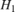and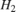such that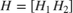, wherean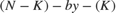submatrix andan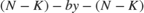submatrix. Let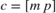be a codeword block, withand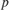representing the information and parity bit sequences, respectively. The encoding consists of these steps.

1. Calculate Alpha Bit Sequence

From the encoding property,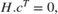,. This calculation of the alpha bit sequence is the same for the IEEE 802.11n/ac/ax and IEEE 802.11ad standards.

2 .Calculate Parity Bit Sequence

The parity bit sequencecan be expressed as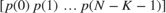. The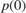term is subsequence of parity bits with the size of a subblock.

For the IEEE 802.11n/ac/ax LDPC base parity-check matrices,is a sparse matrix and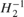has a regular pattern, the array multiplications ofhave linear complexity, which makes it straightforward to compute the parity bit sequence.

For the IEEE 802.11ad LDPC base parity-check matrices,is a sparse matrix andhas an irregular pattern, which makes calculating the parity bit sequence calculation more complicated. Calculating the parity bit sequence consists of solving this equation:So, two separate models were implemented for IEEE 802.11n/ac/ax and IEEE 802.11ad specified LDPC encoders.

IEEE 802.11n/ac/ax Standard LDPC Encoder

The WLAN LDPC Encoder subsystem accepts input data, a control signal, a block length index, and a code rate index. At the start of each frame, the subsystem samples the blockLenIdx and codeRateIdx. The inputController function controls the reading and writing of input data in the Input RAM subsystem and enables the encoding after writing the entire frame to RAM. The Calculate Alpha subsystem calculates the alpha bit sequence and stores its value in the Calculate Alpha/Alpha RAM subsystem. The Calculate Parity subsystem calculates the parity and stores them in the Calculate Parity/Parity RAM subsystem. The outputController function multiplexes and serializes the bits from the Input RAM and Calculate Parity subsystems.

modelName = 'wlan11achdlLDPCEncoder'; load_system(modelName); open_system([modelName '/WLAN LDPC Encoder']); set_param([modelName '/WLAN LDPC Encoder'],'Open','on');Calculate Alpha Bit Sequence

The Calculate Alpha subsystem calculatesbit sequence. Using the parity-check matrix specified in the standard [ 1 ], the ShiftVal LUT and Column LUT blocks calculate and store the shift values for the Circular Shifter subsystem and the address for the Input RAM subsystem. The alphaController function controls the calculation of the alpha bit values by providing the necessary control signals to the Circular Shifter subsystem, ShiftVal LUT block, and Column LUT block. The Circular Shifter subsystem shifts the data circularly, XORs the shifted data with previous shifted data, and stores the data in the Alpha RAM subsystem. By XORing all the alpha values, the model calculates the first column of the parity bit sequence.

set_param([modelName '/WLAN LDPC Encoder'],'Open','off'); open_system([modelName '/WLAN LDPC Encoder/Calculate Alpha']);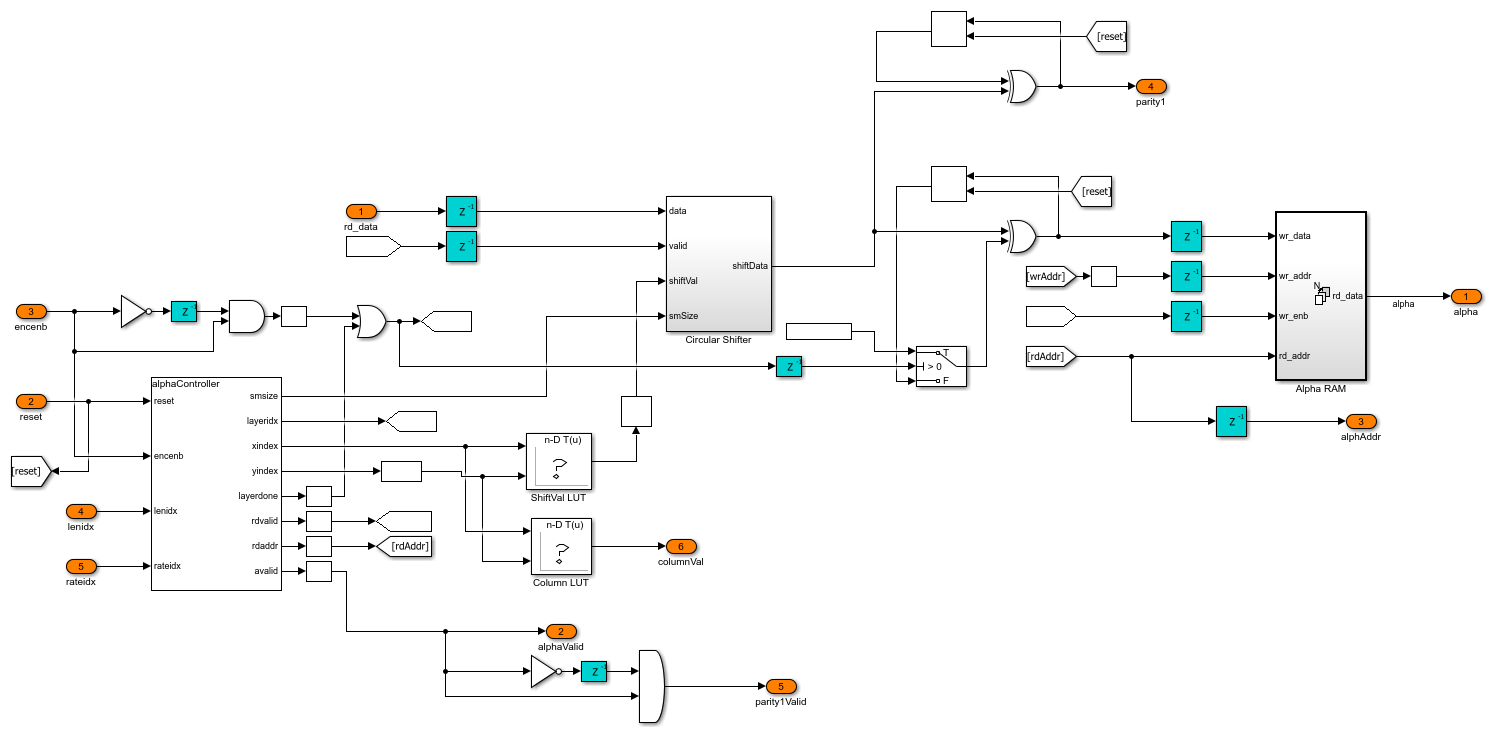Calculate Parity Bit Sequence

The Shiftby1 subsystem shifts the first column of parity bit sequence by one circle. The model then calculates the remaining parity bit subsequences using the shifted first column of parity bit sequence data and the alpha bit values from the Calculate Alpha subsystem. The parityController function controls the parity calculation as well as the reading and writing of parity data to the Parity RAM subsystem.

set_param([modelName '/WLAN LDPC Encoder/Calculate Alpha'],'Open','off'); open_system([modelName '/WLAN LDPC Encoder/Calculate Parity']);The WLAN LDPC Encoder subsystem accepts input data, a control signal, and a code rate index. The subsystem samples the codeRateIdx input at the start of a frame. The architecture is same as the the IEEE 802.11n/ac/ax LDPC Encoder.

modelName = 'wlan11adhdlLDPCEncoder'; load_system(modelName); open_system([modelName '/WLAN LDPC Encoder']); set_param([modelName '/WLAN LDPC Encoder'],'Open','on');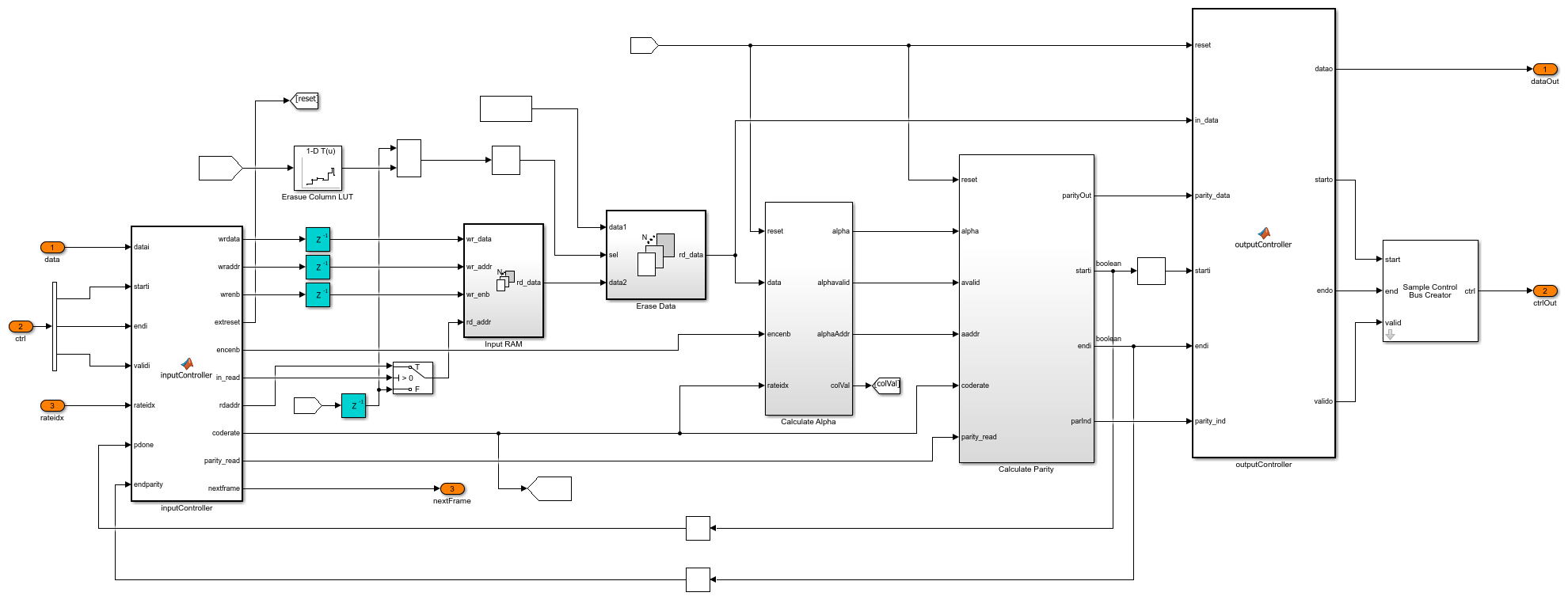Calculate Alpha Bit Sequence

The Calculate Alpha subsystem architecture is same as that of the IEEE 802.11n/ac/ax LDPC Encoder's.

set_param([modelName '/WLAN LDPC Encoder'],'Open','off'); open_system([modelName '/WLAN LDPC Encoder/Calculate Alpha']);Calculate Parity Bit Sequence

The model calculates the first column of the parity bit sequence by XORing the shifted alpha bit values from the Circular Shifter subsystem. Using the first column of parity bit sequence data and the alpha values from the Calculate Alpha subsystem, the parityController function calculates the remaining parity bit subsequences sequentially and stores them in the Parity RAM subsystem. The parityController function controls the parity calculation and writing of parity data to the Parity RAM subsystem. The parityReading function reads the parity data from Parity RAM subsystem and outputs parity bits serially.

set_param([modelName '/WLAN LDPC Encoder/Calculate Alpha'],'Open','off'); open_system([modelName '/WLAN LDPC Encoder/Calculate Parity']);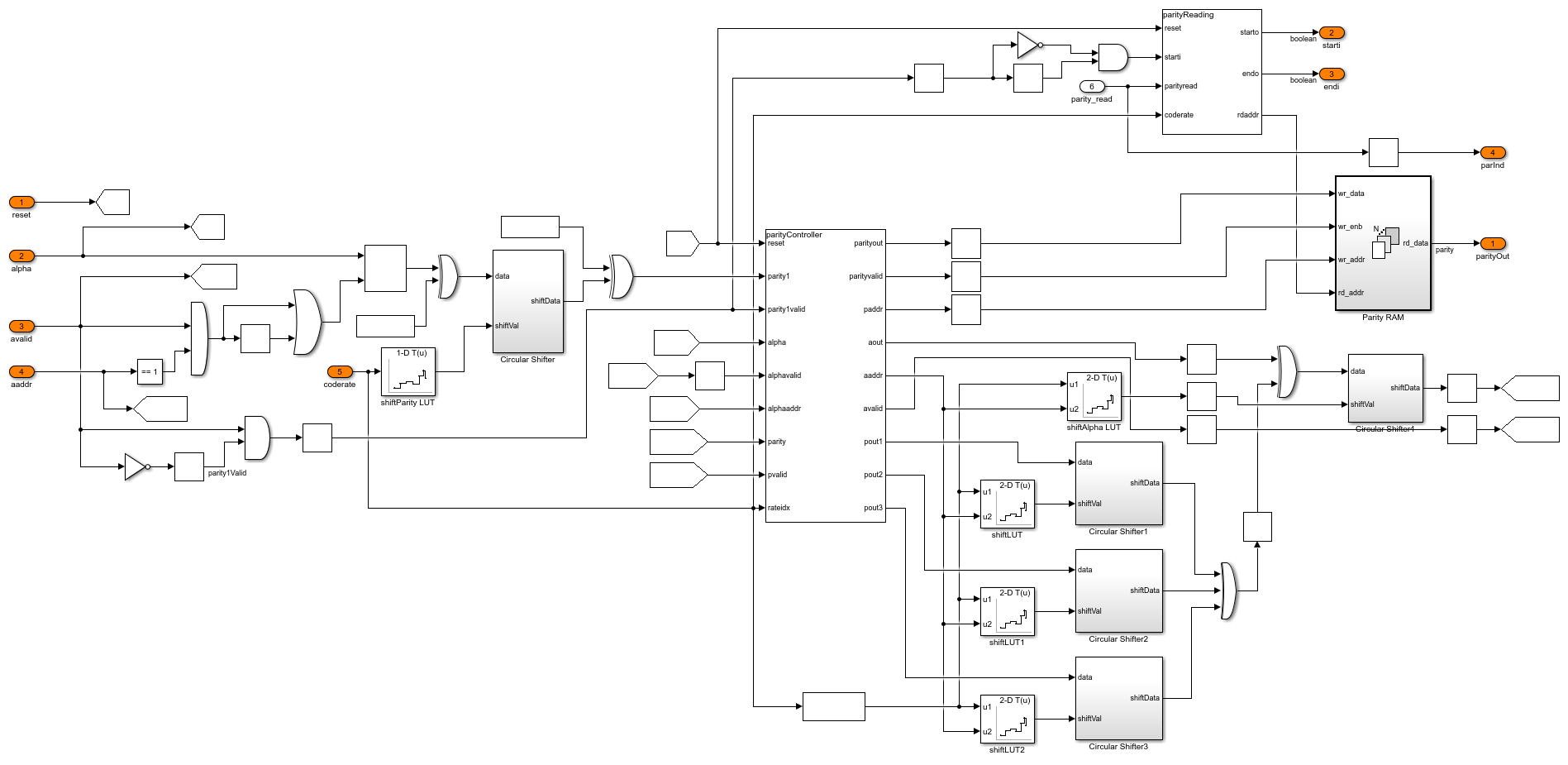### Set Up Input Variables

Choose a WLAN specific standard and input values for the block length and code rate according to your chosen standard. You can change the variable values in this section based on your requirements.

standard = 'IEEE 802.11 n/ac/ax'; % IEEE 802.11 n/ac/ax or IEEE 802.11 ad codeRateIdx = [0; 1; 2; 3]; % Code rate index blkLenIdx = [2; 1; 2; 0]; % Block length index when standard is % set to 'IEEE 802.11 n/ac/ax' numFrames = 4; 

### Generate Input Data

Generate inputs for the ldpcEncode function with the specified block length and code rate variables. Create vectors of block length index and code rate index using the blockLenIdx and codeRateIdx variables, respectively. Convert the frames of input data to samples with a control bus signal that indicates the frame boundaries. Provide these vectors and the control bus as inputs to the WLAN LDPC Encoder subsystem.

The encFrameGap variable accommodates the latency of the WLAN LDPC Encoder subsystem for the specified block length and code rate.

msg = {numFrames}; refOut = cell(1,numFrames); encSampleIn = []; encStartIn = []; encEndIn = []; encValidIn = []; encBlkLenIdxIn = []; encCodeRateIdxIn = []; close_system(modelName); % Calculate input and output codeword length for idx = 1:numFrames if strcmpi(standard,'IEEE 802.11 n/ac/ax') blockLenSet = [648,1296,1944,1944]; rateSet = {'1/2','2/3','3/4','5/6'}; blkLen = blockLenSet(blkLenIdx(idx)+1); codeRate = rateSet{codeRateIdx(idx)+1}; modelName = 'wlan11achdlLDPCEncoder'; else rateSet = {'1/2','5/8','3/4','13/16'}; blkLen = 672; codeRate = rateSet{codeRateIdx(idx)+1}; modelName = 'wlan11adhdlLDPCEncoder'; end % Encoder configuration encConfig = wlanEncoderConfiguration(blkLen,codeRate); % Input bits generation msg{idx} = randi([0 1],encConfig.NumInformationBits,1,'int8'); % LDPC encoding refOut{idx} = ldpcEncode(msg{idx}, encConfig); len = length(msg{idx}); encFrameGap = 2000 + len; encIn = msg{idx}'; encSampleIn = [encSampleIn encIn zeros(size(encIn,1),encFrameGap)]; %#ok<*AGROW> encStartIn = logical([encStartIn 1 zeros(1,len-1) zeros(1,encFrameGap)]); encEndIn = logical([encEndIn zeros(1,len-1) 1 zeros(1,encFrameGap)]); encValidIn = logical([encValidIn ones(1,len) zeros(1,encFrameGap)]); encBlkLenIdxIn = ([encBlkLenIdxIn repmat(blkLenIdx(idx),1,len) zeros(1,encFrameGap)]); encCodeRateIdxIn = ([encCodeRateIdxIn repmat(codeRateIdx(idx),1,len) zeros(1,encFrameGap)]); end dataIn = timeseries(encSampleIn'>0); startIn = timeseries(encStartIn); endIn = timeseries(encEndIn); validIn = timeseries(encValidIn); if strcmpi(standard,'IEEE 802.11 n/ac/ax') blockLenIdx = timeseries(fi(encBlkLenIdxIn,0,2,0)); end codeRateIdx = timeseries(fi(encCodeRateIdxIn,0,2,0)); simTime = length(encValidIn); [columnLUT,shiftLUT] = columnShiftLUTWLAN(standard); 

### Run WLAN LDPC Encoder Model

The WLAN LDPC Encoder subsystem contains the implementation of the WLAN LDPC Encoder block. Run the model to import the input signal variables dataIn, startIn, endIn, validIn, blockLenIdx, codeRateIdx, and simTime to the block from the script. The model exports a stream of encoded output samples encOut and a control bus containing the startOut, endOut, and validOut signals to the MATLAB® workspace.

load_system(modelName); open_system(modelName); sim(modelName);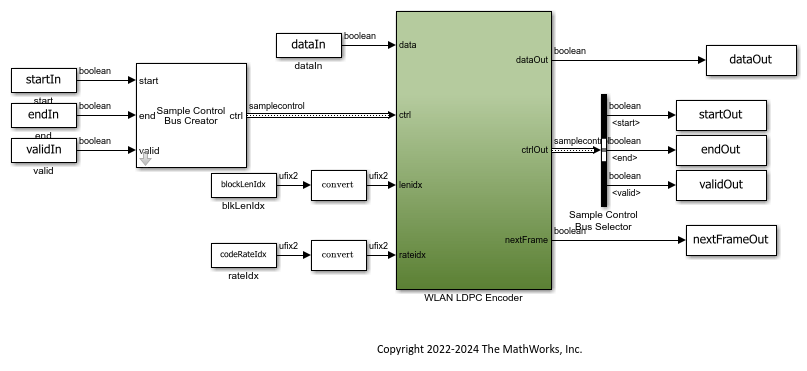### Compare Simulink Block Output with MATLAB Function Output

Convert the streaming data output of the WLAN LDPC Encoder subsystem to frames. Compare the frames with the output of the ldpcEncode function.

startIdx = find(squeeze(startOut)); endIdx = find(squeeze(endOut)); enc = squeeze(dataOut); encHDL = {numFrames}; for i = 1:numFrames idx = startIdx(i):endIdx(i); encHDL{i} = enc(idx); HDLOutput = encHDL{i}; error = sum(abs(double(refOut{i})-HDLOutput(:))); fprintf('Encoded frame %d: Output data differs by %d bits\n',i,error); end 
Encoded frame 1: Output data differs by 0 bits Encoded frame 2: Output data differs by 0 bits Encoded frame 3: Output data differs by 0 bits Encoded frame 4: Output data differs by 0 bits 

Latency

The latency of the WLAN LDPC Encoder block varies with the selected standard, block length, and code rate configurations. This figure shows the latency of the block when you set the blockLenIdx input port to 2 and the codeRateIdx input port to 0.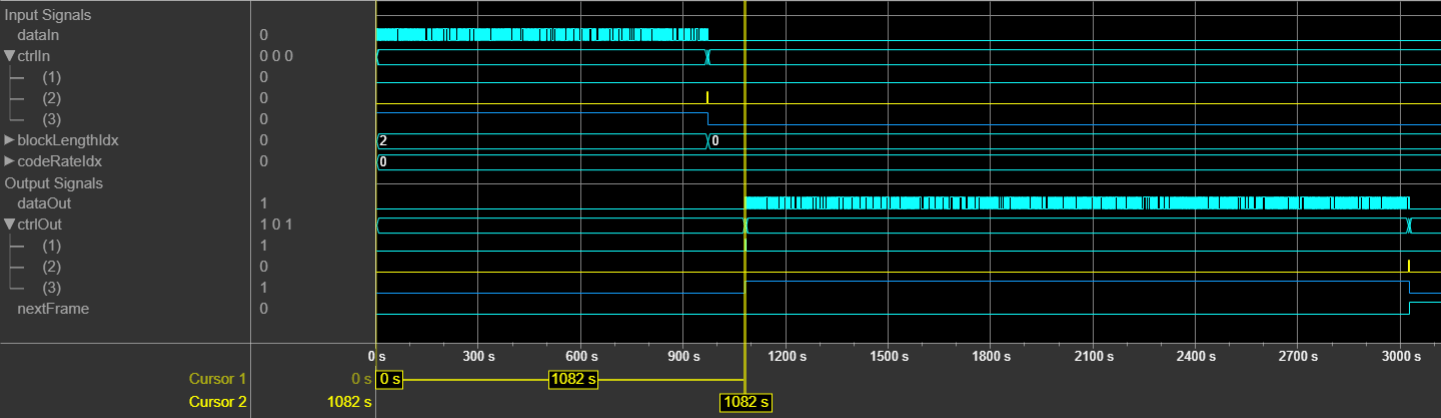This table shows the latency of the WLAN LDPC Encoder block for different standards and configurations.### Generate HDL Code

To check and generate HDL code for this example, you must have an HDL Coder™ license. Use the makehdl and makehdltb commands to generate the HDL code and test bench for the WLAN LDPC Encoder subsystem.

The WLAN LDPC Encoder subsystem is synthesized on a Xilinx® Xilinx Zynq® UltraScale+ MPSoC ZCU102 evaluation board. This table shows the post place and route resource utilization results.

 F = table(... categorical({'IEEE 802.11 n/ac/ax';'IEEE 802.11 ad'}),... categorical({'2495';'2482'}),... categorical({'2153';'1793'}),... categorical({'0.5';'0.5'}),... categorical({'514.88';'516'}),... 'VariableNames',{'Standard','Slice LUTs','Slice Registers','BRAM', ... 'Max. Frequency (MHz)'}); disp(F); 
 Standard Slice LUTs Slice Registers BRAM Max. Frequency (MHz) ___________________ __________ _______________ ____ ____________________ IEEE 802.11 n/ac/ax 2495 2153 0.5 514.88 IEEE 802.11 ad 2482 1793 0.5 516 

### References

1. IEEE Std 802.11™-2020. IEEE Standard for Information Technology - Telecommunications and Information Exchange between Systems - Local and Metropolitan Area Networks - Specific Requirements - Part 11: Wireless LAN Medium Access Control (MAC) and Physical Layer (PHY) Specifications.

2. IEEE Std 802.11ax™-2021. IEEE Standard for Information Technology - Telecommunications and Information Exchange between Systems - Local and Metropolitan Area Networks - Specific Requirements - Part 11: Wireless LAN Medium Access Control (MAC) and Physical Layer (PHY) Specifications - Amendment 1: Enhancements for High-Efficiency WLAN.

3. IEEE Std 802.11ad™-2012. IEEE Standard for Information technology - Telecommunications and information exchange between systems--Local and Metropolitan Area Networks - Specific requirements - Part 11: Wireless LAN Medium Access Control (MAC) and Physical Layer (PHY) Specifications. Amendment 3: Enhancements for Very High Throughput in the 60 GHz Band.